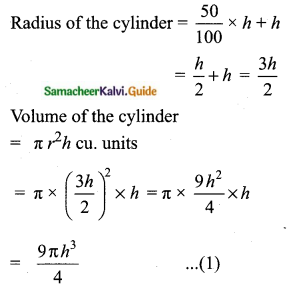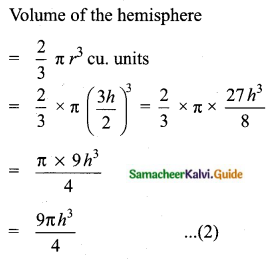Students can download Maths Chapter 7 Mensuration Ex 7.4 Questions and Answers, Notes, Samacheer Kalvi 10th Maths Guide Pdf helps you to revise the complete Tamilnadu State Board New Syllabus, helps students complete homework assignments and to score high marks in board exams.

## Tamilnadu Samacheer Kalvi 10th Maths Solutions Chapter 7 Mensuration Ex 7.4

Question 1.
An aluminium sphere of radius 12 cm is melted to make a cylinder of radius 8 cm. Find the height of the cylinder.
Sphere – Radius r1 = 12 cm
Cylinder – Radius r2 = 8 cm
h2 = ?
Volume of cylinder = Volume of sphere melted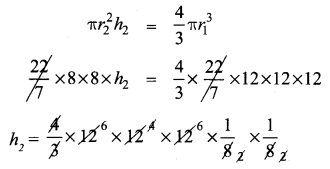∴ Height of the cylinder made = 36 cm.

Question 2.
Water is flowing at the rate of 15 km per hour through a pipe of diameter 14 cm into a rectangular tank which is 50 m long and 44 m wide. Find the time in which the level of water in the tanks will rise by 21 cm.
Length of the rectangular tank (l) = 50 m = 5000 cm
Width of the rectangular tank (b) = 44 m = 4400 cm
Level of water in the tank (h) = 21 cm
Volume of the tank = l × b × h cu. units = 5000 × 4400 × 21 cm3
Radius of the pipe (r) = 7 cm
Speed of the water = 15 km/hr.
(h) = 15000 × 100 cm / hr.
Volume of water flowing in one hour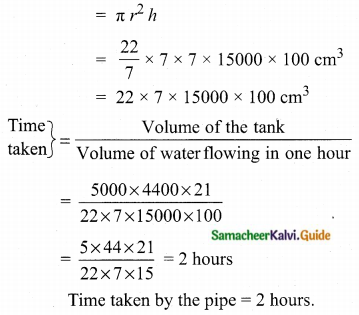Question 3.
A conical flask is full of water. The flask has base radius r units and height h units, the water poured into a cylindrical flask of base radius x r units. Find the height of water in the cylindrical flask.
Height of the conical flask = h units
Volume of the conical flask = $$\frac{1}{3} \pi r^{2} h$$ cu.units
Let the height of the cylindrical flask be “H” units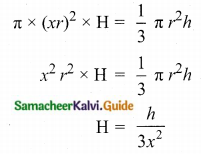Height of the cylindrical flask = $$\frac{h}{3 x^{2}}$$ units

Question 4.
A solid right circular cone of diameter 14 cm and height 8 cm is melted to form a hollow sphere. If the external diameter of the sphere is 10 cm, find the internal diameter.
Radius of a cone (V) = 7 cm
Height of a cone (h) = 8 cm
External radius of the hollow sphere (R) = 5 cm
Let the internal radius be “x”
Volume of the hollow sphere = Volume of the Cone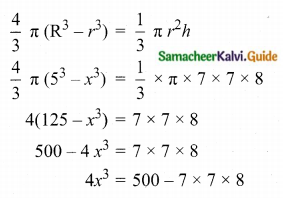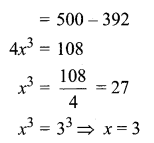Internal diameter of the Hollowsphere = 2 × 3 = 6 cm.

Question 5.
Seenu’s house has an overhead tank in the shape of a cylinder. This is filled by pumping water from a sump (underground tank) which is in the shape of a cuboid. The sump has dimensions 2 m × 1.5 m × 1 m. The overhead tank has its radius of 60 cm and height 105 cm. Find the volume of the water left in the sump after the overhead tank has been completely filled with water from the sump which has been full, initially.
Length of the cuboid tank (l) = 2 cm = 200 cm
Breadth of the cuboid tank (b) = 1.5 cm = 150 cm
Height of the tank (h) = 1 m = 100 cm
Volume of the cuboid = l × b × h cu. units
= 200 × 150 × 100 cm3
= 30,00,000 cm3
Radius of the tank (r) = 60 cm
Height of the tank (h) = 105 cm
Volume of the cylindrical tank = πr2h cu. units
= $$\frac{22}{7}$$ × 60 × 60 × 105 cm3
= 22 × 60 × 60 × 15 cm3
= 1188000 cm3
Volume of water left in the sump = Volume of the sump – Volume of the tank
= 3000000 – 1188000 cm3
= 1812000 cm3

Question 6.
The internal and external diameter of a hollow hemispherical shell is 6 cm and 10 cm respectively. If it is melted and recast into a solid cylinder of diameter 14 cm, then find the height of the cylinder.
Internal radius of the shell (r) = 3 cm
External radius of the shell (R) = 5 cm
Radius of the cylinder (r) = 7 cm
Let the height of the cylinder be “h”
Volume of the cylinder = Volume of the hemispherical shell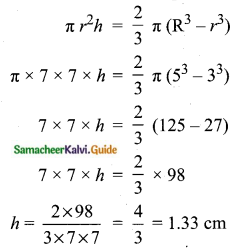Height of the cylinder = 1.33 cm

Question 7.
A solid sphere of radius 6 cm is melted into a hollow cylinder of uniform thickness. If the external radius of the base of the cylinder is 5 cm and its height is 32 cm, then find the thickness of the cylinder.
Radius of a sphere (r) = 6 cm
External radius of the cylinder (R) = 5 cm
Height of the cylinder (h) = 32 cm
Let the internal radius of the cylinder be ‘x’
Volume of the hollow cylinder = Volume of a sphere
πh (R2 – r2) = $$\frac{4}{3}$$ πr3
π × 32 (5 + x) (5 – x) = $$\frac{4}{3}$$ × π × 6 × 6 × 6
32 (25 – x2) = 4 × 2 × 6 × 6
25 – x2 = 9
x2 = 25 – 9 = 16
x = √16 = 4
Thickness of the cylinder = 5 – 4 = 1 cm.

Question 8.
A hemispherical bowl is filled to the brim with juice. The juice is poured into a cylindrical vessel whose radius is 50% more than its height. If the diameter is same for both the bowl and the cylinder then find the percentage of juice that can be transferred from the bowl into the cylindrical vessel.